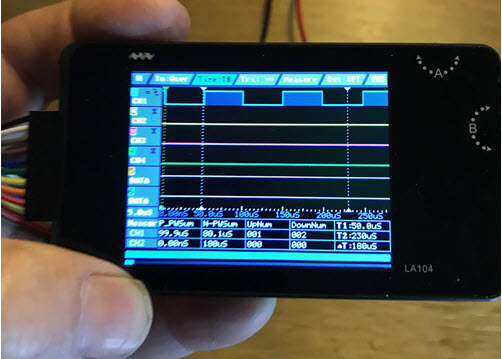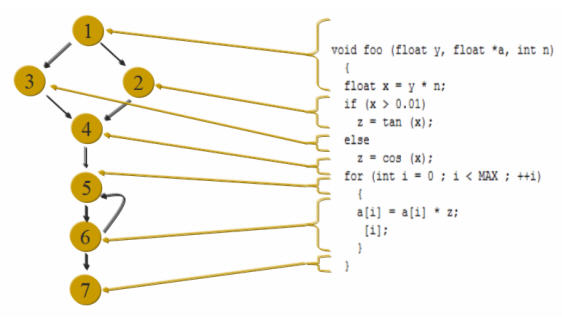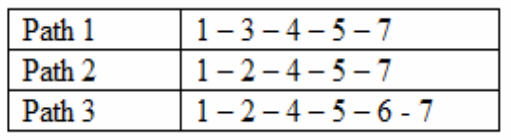For novel ideas about building embedded systems (both hardware and firmware), join the 40,000+ engineers who subscribe to The Embedded Muse, a free biweekly newsletter. The Muse has no hype and no vendor PR. Click here to subscribe.October's giveaway is a LA104 mini logic analyzer. I reviewed it in Muse 406. .Enter the contest here.

# Basis Path Testing

Published on embedded.com, October 2012

Few people bother to measure anything in the firmware world, which is a shame. For this to be engineering we need to apply known principles, measure the results, and use that data to improve our systems.

For instance, is there a metric that tells us anything about how many tests we should do on a particular function?

But there are other, less onerous, metrics that are useful, if imperfect. For instance, Tom McCabe invented the notion of cyclomatic complexity. That's an integer that represents the complexity of a function (not of the program; it's measured on a per-function basis). It's a simple notion: cyclomatic complexity (for some reason always expressed as v(G)) is the number of paths through a function. A routine with 20 assignment statements has a complexity of 1. Add a simple if statement and it increments to two.

A more formal definition uses the concept of "nodes" and "edges." A node is one direction the code can go. An edge is the connection between nodes. V(G) is:

`v(G) = edges -- nodes + 2`

It's easier to visualize this graphically. Each of the circles in the following diagram is a node; the arrows connecting them are edges:Running the math (or tracing the paths through the code) and we see that v(G) is 3.

The complexity is the minimum number of tests one must run in order to insure a function is fully tested. In this example, two tests are provably insufficient. In other words, cyclomatic complexity is a metric that gives us a lower bound on the efficacy of the test regime. It says nothing about the maximum number needed, which may be a lot higher for complex conditions. But at least it gives us a bound, a sanity check to insure our tests don't fall below the minimum needed.

One way to address this is to make a table of all possible paths, which for this example looks like (the numbers are the nodes from the previous diagram that the code flows through):Build the table and compare it against v(G); if the number of paths identified in the table isn't at least v(G) than the table is incomplete. This is a beautiful thing; it's a mathematical model of our testing.

A lot of tools will automatically do this for you; some will even create the edge/node diagram and, based on that, emit the C code needed to do the testing (Examples include those from LDRA and Parasoft). Isn't it amazing that, given that it's so hard -- and expensive - to get testing right, that so few developers use these tools?

Sure, the tools cost money. So let's do the math. Suppose a system has 100 KLOC. If the developers follow the rules and limit functions to a page of code in length -- let's say 50 lines -- that's at least 2000 functions. Most pundits recommend limiting the complexity of a function to somewhere between 10 and 15. To be conservative we'll assume the average complexity is 5. That means we need at least 10,000 tests.

In the US the loaded cost of an engineer is \$150k - \$200k/year. Let's assume the former. That means the engineer costs his company \$75/hour or \$1.25/minute. If we have a Spock-like programmer who can create the diagram above, parse it, figure out a test, code it, get it to compile correctly, link, load and run the test in just one minute, and sustain that effort for 10,000 minutes (166 hours, or a work-month) without even a bathroom break, those tests cost \$12.5K. Substitute in real numbers and the result becomes horrifying.

The tools start to look pretty darn cheap.

Alternatively, if you don't use the tools and construct the graphs manually, then you're forced to really look at the code. And in the example above there are at least two bugs that quickly become apparent. So the quality goes up before testing begins.

(Of course, there are plenty of tools that would identify those bugs, too).